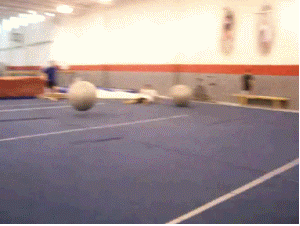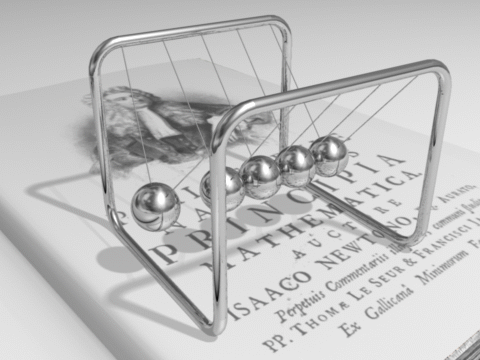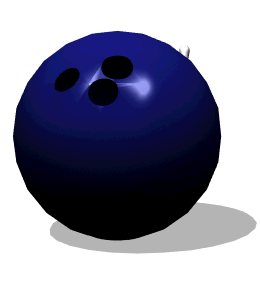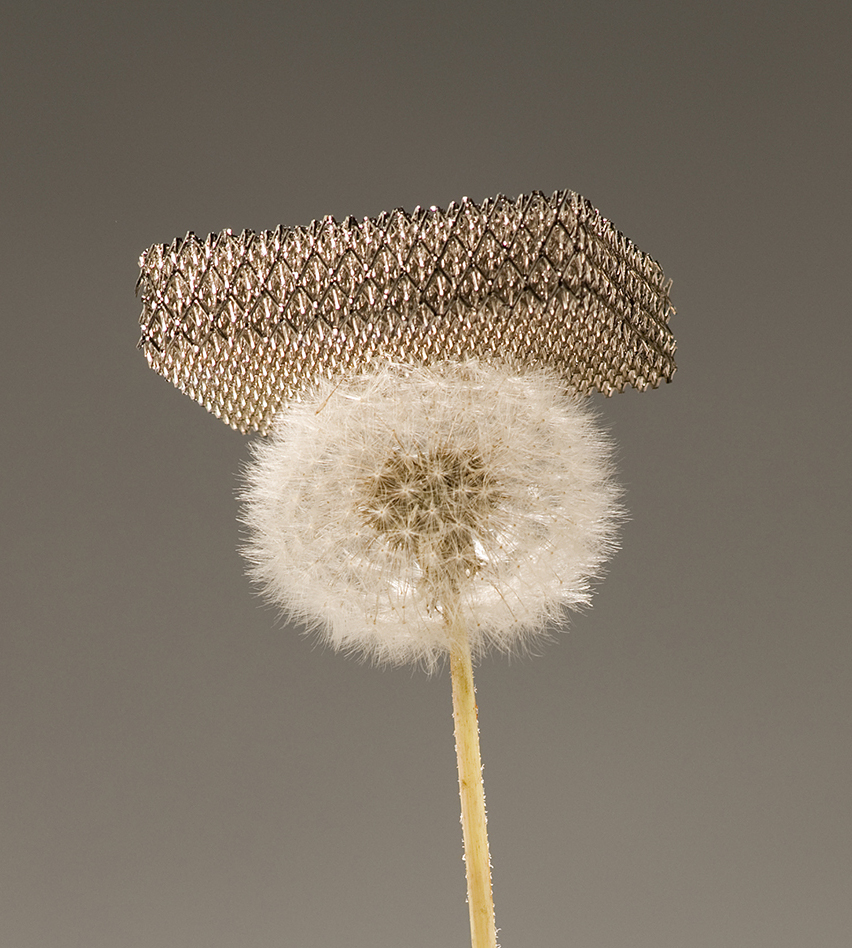HomeTry This At Home

Review - Explain this using one of Newton's Laws

A lead brick has a lot of mass
and therefore a lot of inertia.

The greater the mass, the greater the tendency for an object to remain at rest, the smaller the acceleration that is produced by a given force.Show Me The Physics

Momentum Word Problems

 J = Ft = mΔv = Δp

 Ex 1)  A 5.0 kg mass has its velocity change from 8.0 m/s east to 2.0 m/s east.  Find the objects change in momentum.

Ex 1)  A 5.0 kg mass has its velocity change from 8.0 m/s east to 2.0 m/s east.  Find the objects change in momentum.

m = 5.0 kg
Vi = 8.0 m/s East
Vf = 2.0 m/s East
Δp = ?

 J = Ft = mΔv =Δp

Δp = mΔV

= (5.0 kg)(2.0 m/s - 8.0 m/s)

= -30. kgm/s East

or +30. kg m/s WestEx 2) A 5.0 kg mass moving with a velocity of 8.0 m/s east has an impulse applied to it which causes its velocity to change to 20. m/s East.   Find Impulse:

Ex 2) A 5.0 kg mass moving with a vector of 8.0 m/s east has an impulse applied to it which causes its velocity to change to 20. m/s East.

Find Impulse:

m = 5.0 kg
Vi = 8.0 m/s East
Vf = 20. m/s East
J = ?

 J = Ft = mΔv =Δp

J = mΔv = (5.0 kg)(12. m/s East)

= 60. kg m/s east

= 60. Ns East

Find the force if the impulse was applied for 3.0 sec.

F = ?

t = 3 seconds

m = 5.0 kg

Vi = 8.0 m/s East

Vf = 20. m/s East

J = 60. kg m/s east

 J = Ft = mΔv =Δp

J = Ft = 60. Ns East

F(3.0 sec) = 60. Ns East

F = 20. N East

Golf Ball hitting steel super slo mo

GolfBallhittingsteelsuperslomo.mp4

 Ex 3) How long would it take for a net upward force of 100. N, to increase the speed of a 50. kg object from 100. m/s to 150. m/s.

Ex 3) How long would it take for a net upward force of 100. N, to increase the speed of a 50. kg object from 100. m/s to 150. m/s.

F = 100. N

m = 50. kg

Vi = 100. m/s

Vf = 150. m/s

t = ?

 J = Ft = mΔv =Δp

FΔt  = mΔv

(100. N)t  =  50. kg(50. m/s)

t  =  25. secs

 Ex 4)  A 1.0 kg ball traveling @ 4.0 m/s strikes a wall and bounces straight back @ 2.0 m/s. Find ΔpRacquetball Slow-Mo - HiViz

Ex 4)  A 1.0 kg ball traveling @ 4.0  m/s strikes a wall and bounces straight back @ 2.0 m/s

Find Δp

m = 1.0 kg

Vi = 4.0 m/s

Vf = ?

Vf = -2.0 m/s

(opposite direction)

Δp = ?J = Ft = mΔv =Δp

(a) Δp = mΔv

= (1.0 kg)(-2.0 m/s - 4.0 m/s)

= - 6.0 kgm/s

(b) What is impulse applied to the ball?

J =  Δp = -6.0 kgm/s

(c) What is impulse applied to the wall?

J = +6.0 kgm/s

3rd Law, Action ReactionComputer animation of a Newtons' cradle

DemonDeLuxe (Dominique Toussaint)World's Lightest Material
200 times lighter than styrofoam

HRL Laboratories - See the video

Using an innovative fabrication process developed at HRL, researchers created a “micro-lattice” structure of interconnected hollow tubes with a wall thickness of 100 nanometers, 1,000 times thinner than a human hair.
Download high resolution image. High resolution video is available upon request by emailing media@hrl.com.
Photo credit: Photo by Dan Little © HRL Laboratories,

AP Physics

p. 188) 17

Dp = mDv

Momentum only changes in the x direction

toward wall +

Vi = Vsinq

Vf = -Vsinq

Dp = mDv

Dp = m(-2Vsinq)

= 2.1 kgm/s left

Review

 A teacher pushed ice cubes of different masses with different amounts of force. Which diagram shows the cube that will show the biggest velocity change when pushed with the force represented by the arrow?A) A B) B C) C D) D E) To find the velocity change, you need the force's contact time.[Law Of ConservationOf Momentum]